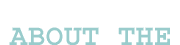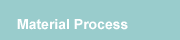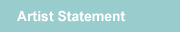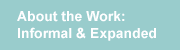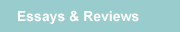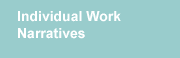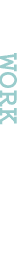Identity Sequence Pascal’s Triangle Green My first doodles of Pascal’s triangle on graph paper revealed an architectural form that I found irresistibly beautiful, almost sexy. The triangle form was reminiscent of a bridge, an architecture I equate with building of civilizations and society. When I coupled two of the bridge forms in a mirror relationship, I saw a small section of a strand whose form could speak to an internal architecture or code of some sort of organic entity, a microscopic code enlarged. Identity Sequence Pascal’s Triangle Green is based on digits from the first six rows of Pascal's Triangle. Blocks of the same color represent each digit. 2 panels which make 1 rooftop form equal 1 unit, so 5 ochre rooftop forms represent digit 5, etc. Two triangles, one inverted, touch at their beginning digit. Bottom triangle is constructed from 5x7 inch panels, the top from 5x5s. Panels are joined at approximately 90 degrees so that each row looks like an unfolded folded-book. Also, focusing on how the material process parallels identity construction, I crocheted all the units in this group to be the same. Each single unit used the same stitch and the same number of stitches to produce a mass of individuals who began exactly the same but by enduring the steps within this material process, the cookie cutter units took baby steps towards individuation. There are many opportunities within each process step to lose control of the form and distort each unit in its own unique way. Upon first look, the small units that assemble to create the one large body seem the same, but in actuality, no two units are. Brief definition of Pascal’s Triangle Pascal’s Triangle is a big triangle of numbers. The two major areas where Pascal’s Triangle is used are Algebra and Probability where it can be used to find combinations. Triangular numbers and the Fibonacci numbers can also be found in Pascal’s triangle. 1 1   1 1   2    1 1    3    3    1 1    4   6    4    1 1   5   10   10   5    1 Start out with the top two rows as 1, and 1 1. To construct the next line down, look at the two entries above it, the one above it and to the right and the one above it and to the left, and then place 1 at the far right and 1 at the far left. For example, if constructing the line below ( 1 3 3 1 ), ( 1+3=4, 3+3=6, 3+1=4 ), add the 1 at each end and you have ( 1 4 6 4 1 ).